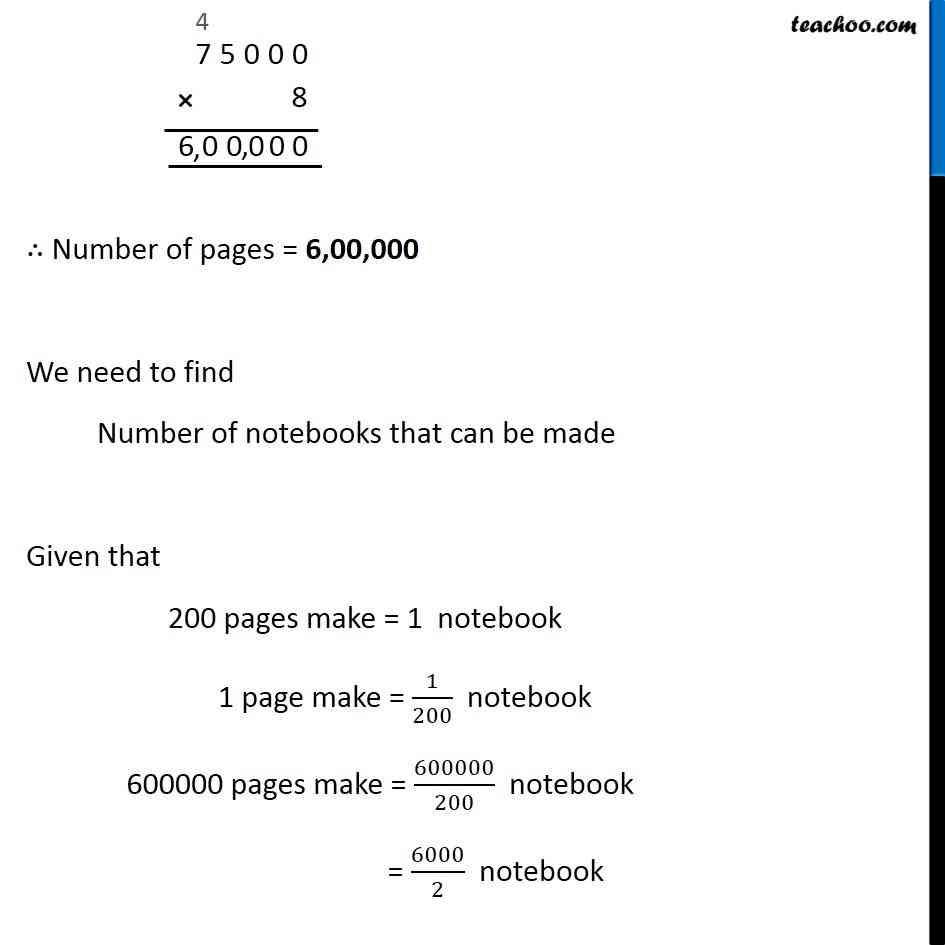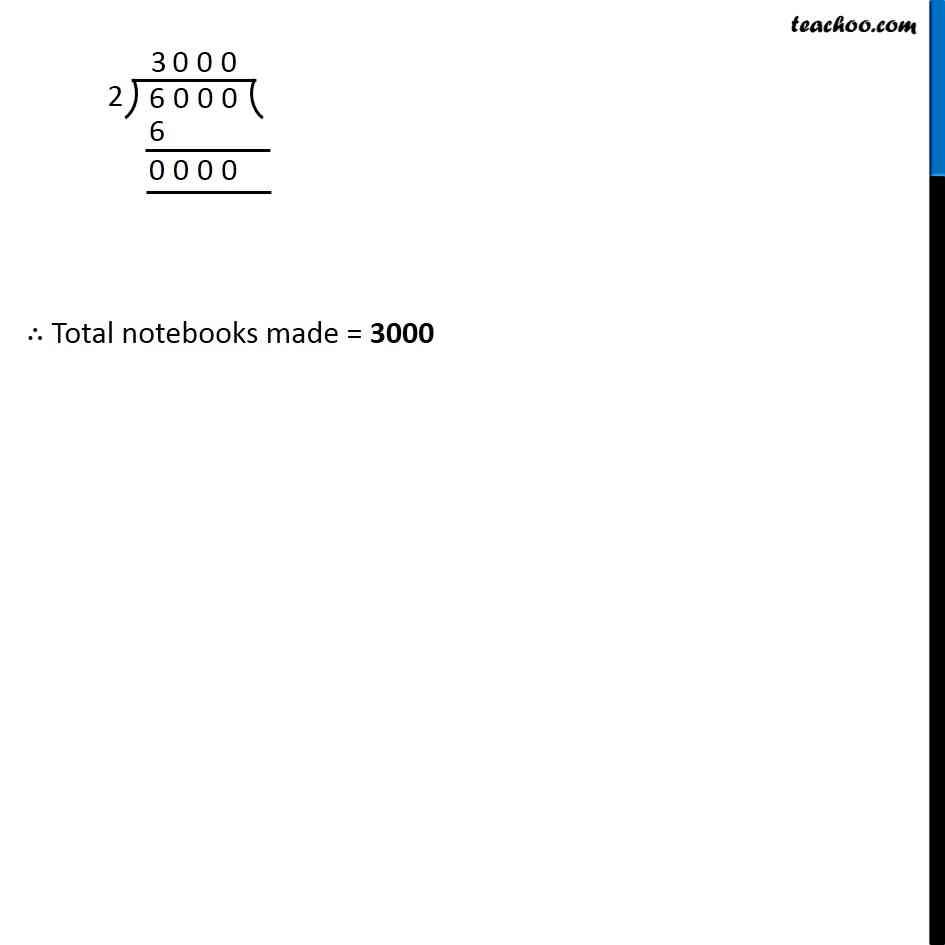Subscribe to our Youtube Channel - https://you.tube/teachoo

1. Chapter 1 Class 6 Knowing our Numbers
2. Concept wise
3. Division of large numbers

Transcript

Example 4 The number of sheets of paper available for making notebooks is 75,000. Each sheet makes 8 pages of a notebook. Each notebook contains 200 pages. How many notebooks can be made from the paper available? Total sheets = 75000 Given that, Number of pages from 1 sheet = 8 Number of pages from 75000 sheets = 75000 × 8 ∴ Number of pages = 6,00,000 We need to find Number of notebooks that can be made Given that 200 pages make = 1 notebook 1 page make = 1/200 notebook 600000 pages make = 600000/200 notebook = 6000/2 notebook ∴ Total notebooks made = 3000

Division of large numbers